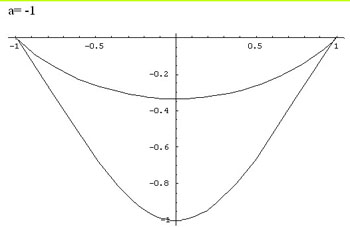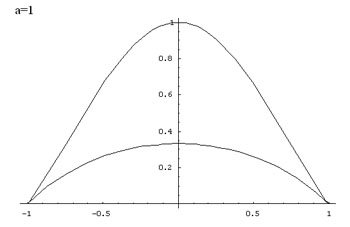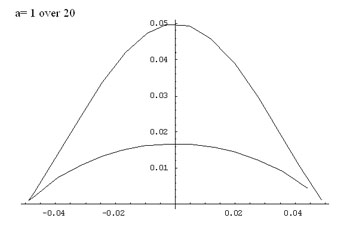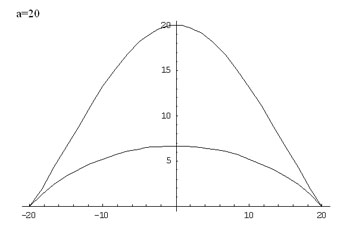#### You may also likeFind all real solutions of the equation (x^2-7x+11)^(x^2-11x+30) = 1.### Kissing

Two perpendicular lines are tangential to two identical circles that touch. What is the largest circle that can be placed in between the two lines and the two circles and how would you construct it?### Good Approximations

Solve quadratic equations and use continued fractions to find rational approximations to irrational numbers.

# Cocked Hat

##### Age 16 to 18 Challenge Level:

 Congratulations Aleksander from Gdynia Bilingual High School No3, Poland for your excellent solution to the Cocked Hat problem. As you will see, the solution hinges on simplification of an algebraic expression and solving a quadratic equation.

Here is Aleksander's solution.
First we will rearrange the expression from implicit to explicit form. The given equation is

$$(x^2 + 2ay -a^2)^2 = y^2(a^2 - x^2)$$

Squaring the LHS gives

$$4a^2y^2 + 4ay(x^2-a^2) + (x^2 - a^2)^2 = y^2(a^2 - x^2).$$

Collecting like terms gives the quadratic equation

$$y^2(3a^2+x^2)+ 4ay(x^2-a^2) + (x^2 - a^2)^2 = 0.$$

The discriminant is

$$\Delta = {16a^2(x^2 - a^2)^2-4(3a^2+x^2)(x^2-a^2)^2}= (x^2 - a^2)^2(4a^2-x^2) = 4(a^2 - x^2)^3.$$

Solving this equation we get:

\eqalign{ y &= {-4a(x^2 - a^2)\pm \sqrt{4(a^2 - x^2)^3}\over 2(3a^2+x^2)} \cr &= {(x^2-a^2)(-2a\pm \sqrt{(a^2-x^2)})\over (3a^2+x^2)} }

For each value of $a$ there are two branches of the graph given by taking the +ve and -ve signs in this equation. Values of $y$ are only defined for the interval $-a \leq x \leq a$. The domain of $f(x)$ is $-a \leq x \leq a.$ The zeros of the function are given by $x = a$ and $x = -a.$

Additionally, the function is symmetric with respect to the y-axis, because $x$ always appears in even powers. Graphs for parameters $a = n$ and $a = -n$ and are symmetric to each other with respect to the x-axis, that is $y(n) = y(-n)$. Proof:

$${(x^2-n^2)(-2n\pm \sqrt{(n^2-x^2)})\over (3n^2+x^2)} = -{(x^2-(-n)^2)(-2(-n)\pm \sqrt{(-(n)^2-x^2)})\over (3(-n)^2+x^2)}$$

Here are some graphs of the function: